# Representing Fractions By Partitioning a Number Line

Visual math talk prompts used to help students better conceptualize fractions by partitioning a number line into different fractional amounts.

## In This Set of Math Visual Prompts…

Students will explore representing, comparing and ordering fractions by partitioning a 0-2 number line.

## Intentionality…

This set of visual math talk prompts is taken from the Math Talk section of Day 2 in the Make Math Moments Problem Based Unit called Wooly Worm Race. The purpose of the Math Talk is to reinforce key concepts and big ideas from this problem based math unit including:

• Fractions can be represented in a variety of ways;
• How you partition the whole determines the fractional unit (i.e.: partitioning a whole into 6 parts will result in 6 sixth parts);
• As you partition a whole into more parts, the smaller the size of each part;
• As you partition a whole into more parts, the larger the denominator;
• In order to compare two or more fractional quantities, the whole must be the same;
• The numerator indicates the number of parts relative to the number of parts in the whole indicated by the denominator; and,
• Fractional amounts exist between whole numbers.

Unsure about some of the terminology listed above?

## Preparing to Facilitate

In today’s visual math talk, students will be asked to represent different fractional amounts on a number line from 0 to 2. The goal of this visual math talk is to build student flexibility and fluency with fractional quantities while also practicing partitioning a linear model.

The prompt for each problem in this visual math talk is:

Where would you place each fractional amount on the number line?

Consider adding context by exploring the first day of the Wooly Worm Race problem based lesson where we can frame the 0 to 2 number line to represent the 2 metre track that the caterpillars would race along during that particular lesson.

Introduce each quantity, one at a time, for students to place on a number line.

one

one half

¼

⅛

6/8

Encourage students to draw a new number line from 0 to 2 for each prompt in order to promote additional practice of partitioning as well as to ensure they do not get confused with partitions that were helpful for one prompt, but not so helpful for another.

Three (3) additional visual math prompts as well as modifiable purposeful practice problems shared via Google Docs and/or Microsoft Word can be found in the Teacher Guide from Day 2 of the Wooly Worm Race problem based math unit

Although you could lead this math talk by orally sharing the context and representing student thinking on a chalkboard/whiteboard, consider leveraging the following visual math talk prompt videos to ensure accessibility for all students. We would recommend that you still model student thinking to ensure student voice is honoured prior to sharing the visual silent solution shared in the visual prompt video.

Visual Math Talk Prompt #1

Begin playing the video to share the first visual math talk prompt and be ready to pause the video in order to give students time to think.

The prompts are simple and shared without context, however since this is taken from Day 2 of the Wooly Worm Race problem based math unit, you might consider leveraging the context from the previous day involving caterpillars racing along a 2 metre straight track. In that case, you could verbally share the context using a script such as:

The Wooly Worms are racing in their next competition!

Woolzy managed to race 1 metre.

Where on the track from 0 metres to 2 metres did Woolzy finish?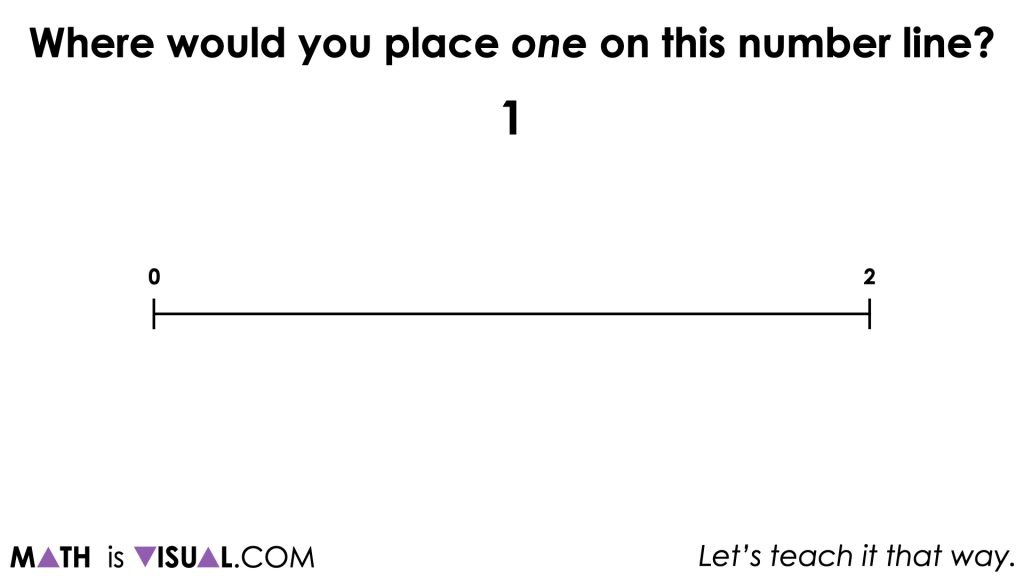In this first prompt, students will likely have no problem partitioning the 0 to 2 number line into two parts and indicating a value of 1 at the halfway point.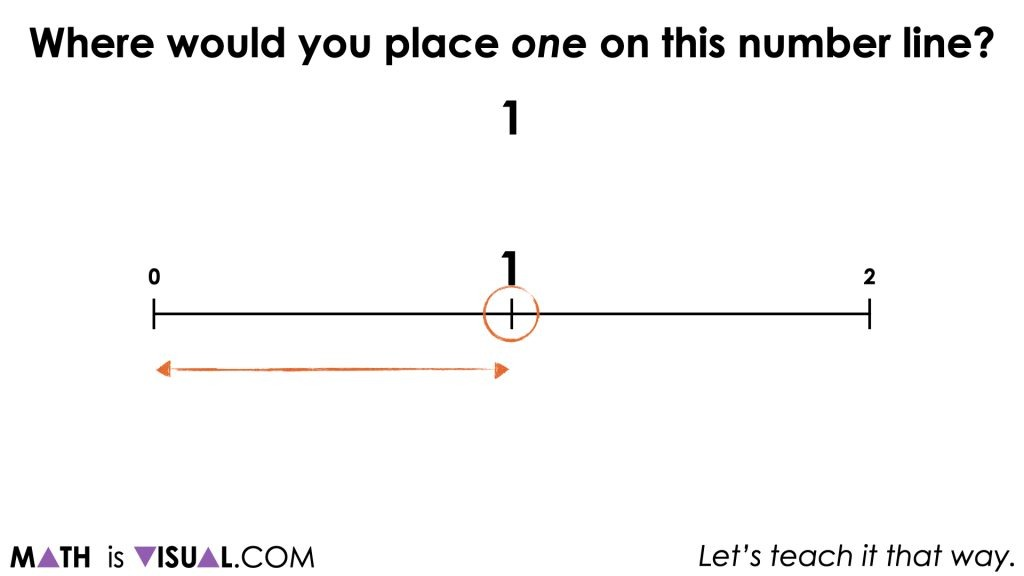Visual Math Talk Prompt #2

Continue playing the video until prompted to pause and consider leveraging the Wooly Worm context describing where the second caterpillar finished the race at the 1 half metre mark.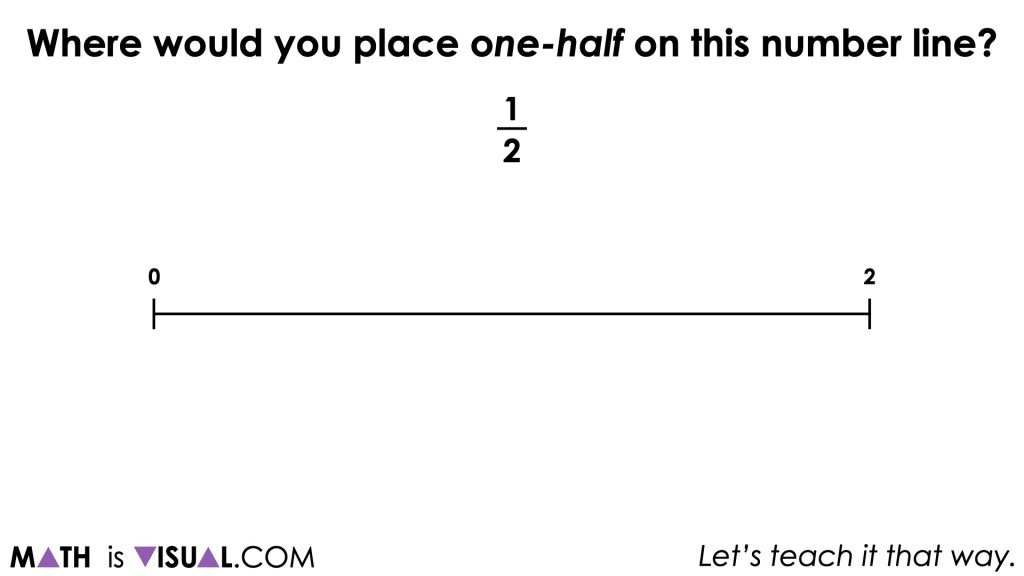Encouraging students to draw another number line rather than simply building on the first number line will give them the opportunity to (likely) partition the number line in half and then partition each half in half.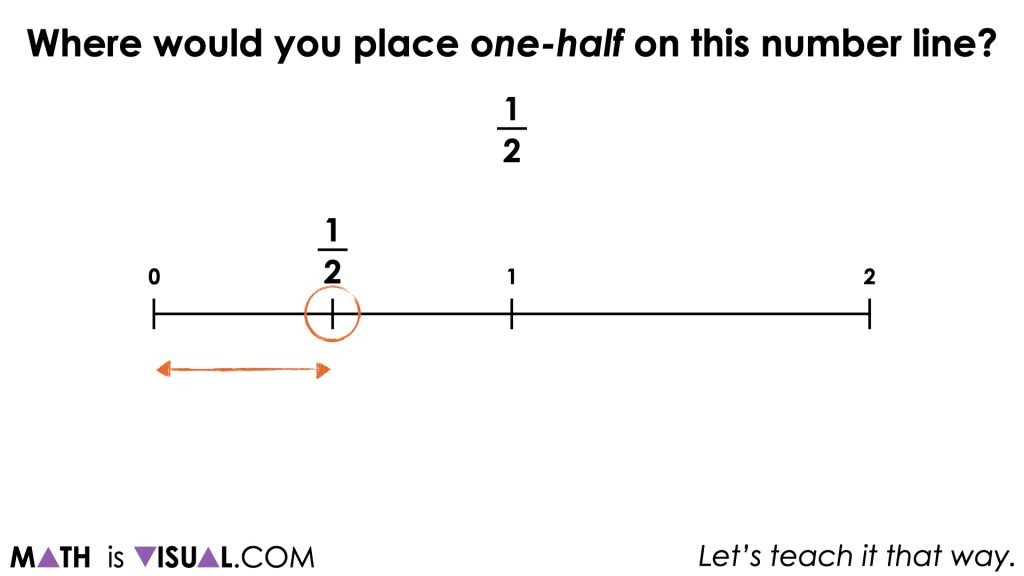Depending on the readiness of the learners, you might consider mentioning that we have essentially taken one half of one half which can be written symbolically as ½ x ½ to emerge the idea of multiplying fractions.

Visual Math Talk Prompt #3

Continue playing the video until prompted to pause and consider leveraging the same context verbalizing something similar to:

Show where the third caterpillar finished the race at the ¼ metre mark on the 2 metre long track.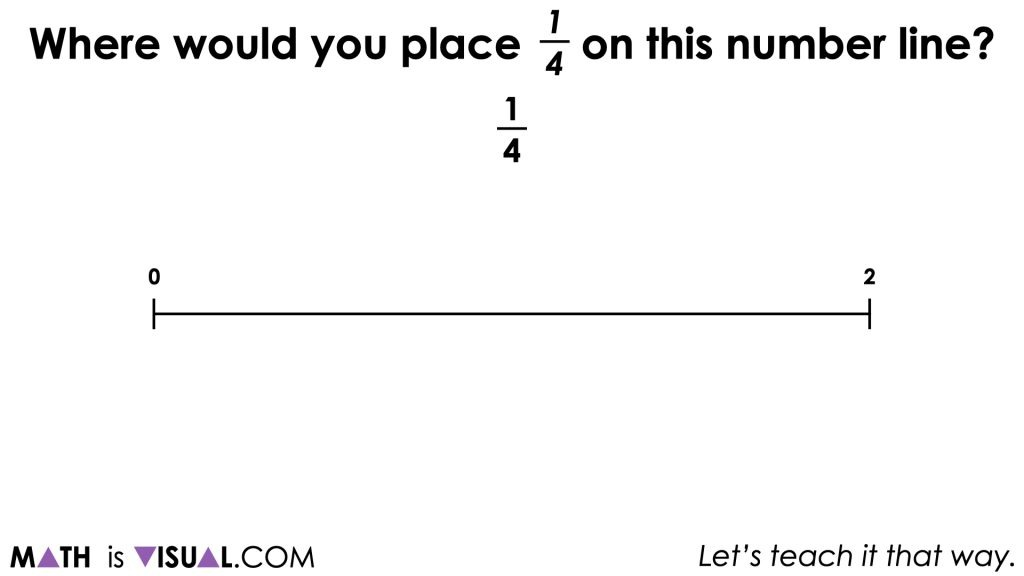Again, it is nice to encourage students to draw another number line for this prompt, however they can ultimately decide how they choose to model their thinking.

In this case, students are halving the 0-2 number line, then halving those resulting parts and then finally, halving those parts again to reveal ¼ on the 0-2 number line.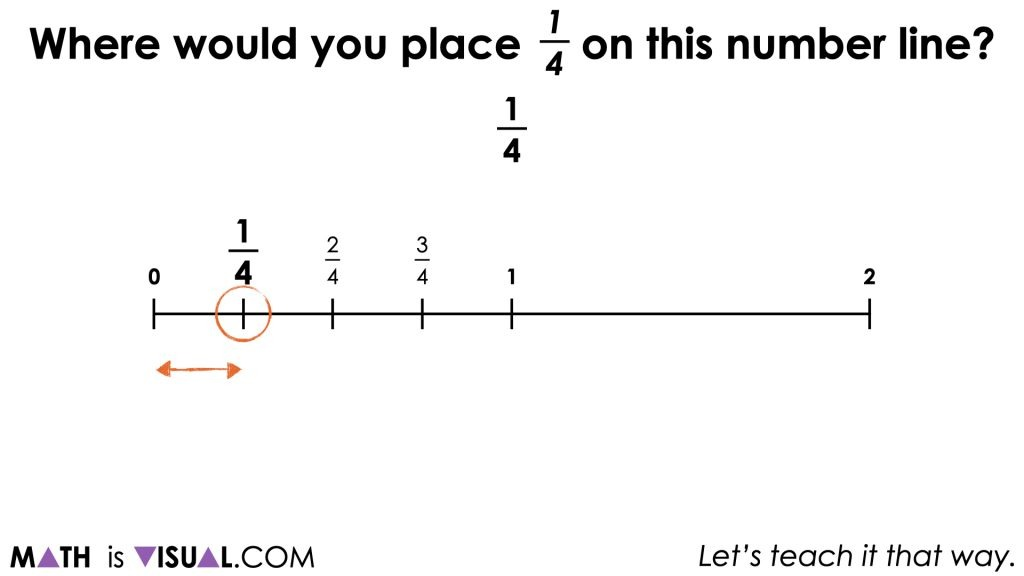There are a number of different ways we could represent this symbolically. For example, we could say that we took half of a half of a half of the 0-2 number line:

½ of ½ of ½ of 2

= ½ x ½ x ½ x 2

= ½ x ½ x (½ x 2)

= ½ x ½ x (1)

= ½ x (½ x 1)

= ½ x (½)

= ½ x ½

= ¼

Is this symbolic representation a bit of overkill? Absolutely. However, if this helps students better connect multiplying fractions symbolically to the act of partitioning our number line repeatedly, then it could be worth the while.

Visual Math Talk Prompt #4

Continue playing the video until prompted to pause and consider leveraging the same context verbalizing something similar to:

Show where the third caterpillar finished the race at the ⅛  metre mark on the 2 metre long track.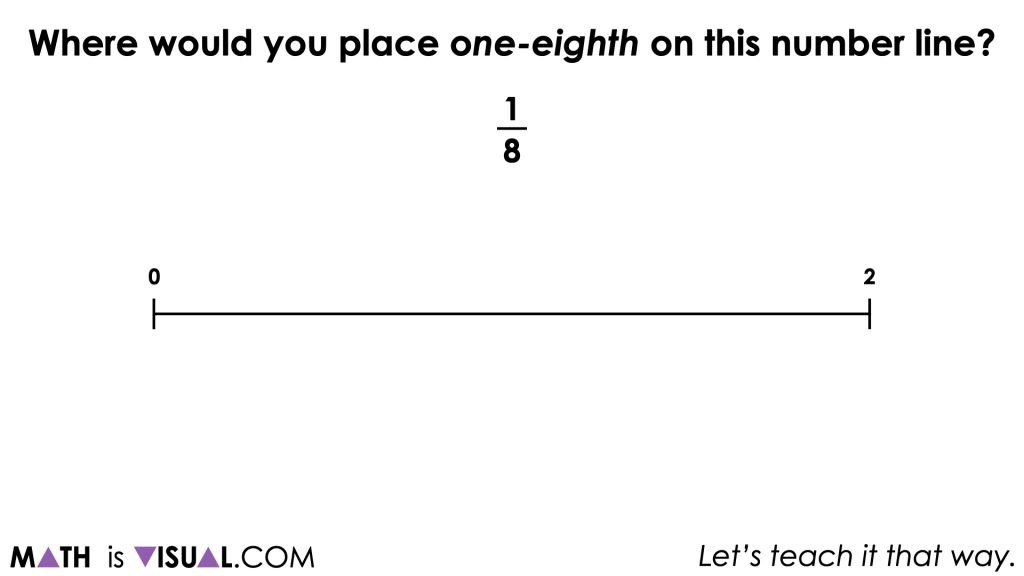Again, we are going to be encouraging students to use a new 0 to 2 number line and begin the process of partitioning to determine where 1 eighth would be placed on the number line.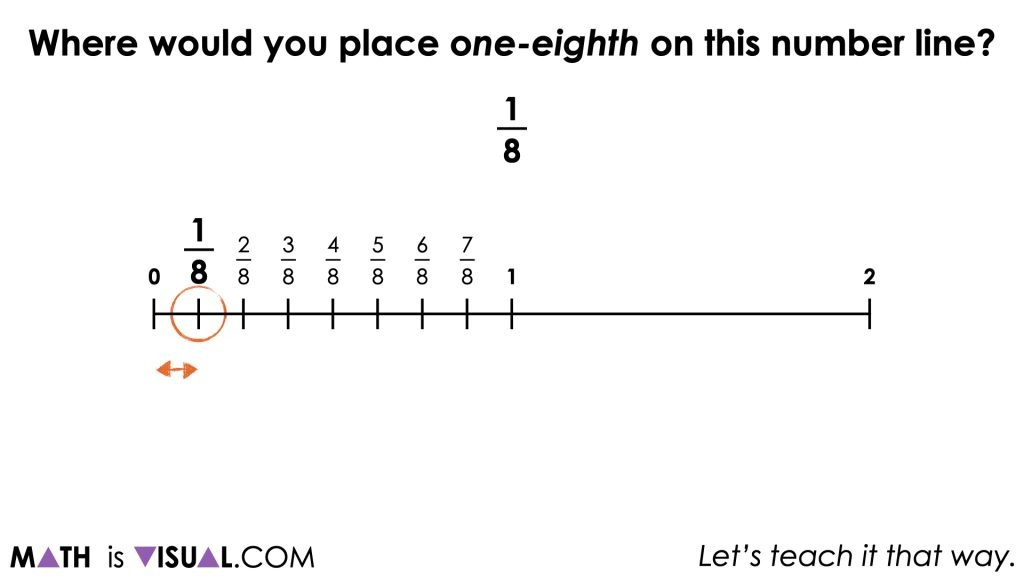Consider asking students to elaborate on what they have ultimately done to determine 1 eighth of a metre.

Visual Math Talk Prompt #5

Continue playing the video until prompted to pause and consider leveraging the same context verbalizing something similar to:

Show where the third caterpillar finished the race at the 6/8 metre mark on the 2 metre long track.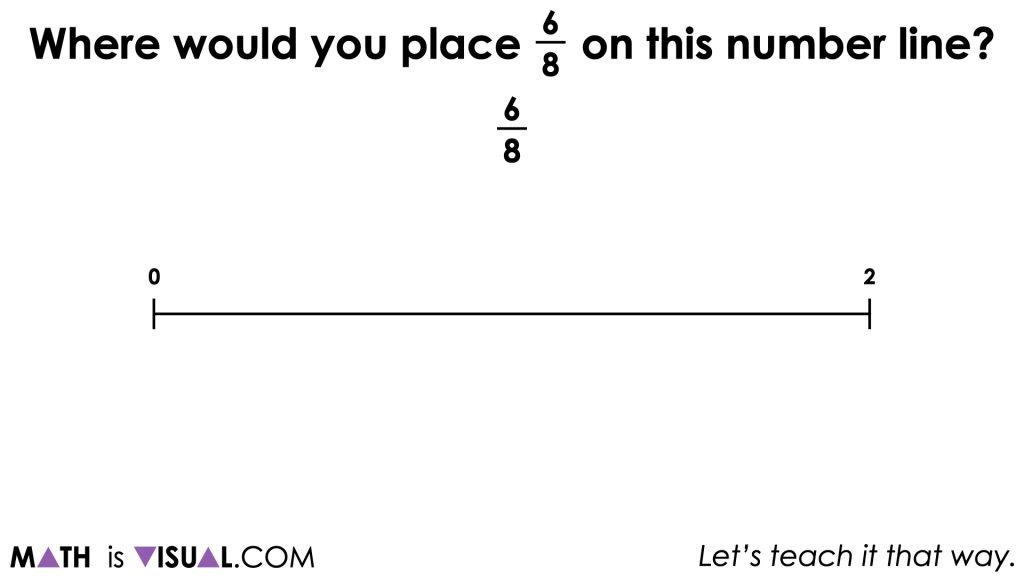With this particular prompt, you might not require students to re-partition a brand new number line, but rather to articulate where 6 eighths would be placed on the number line.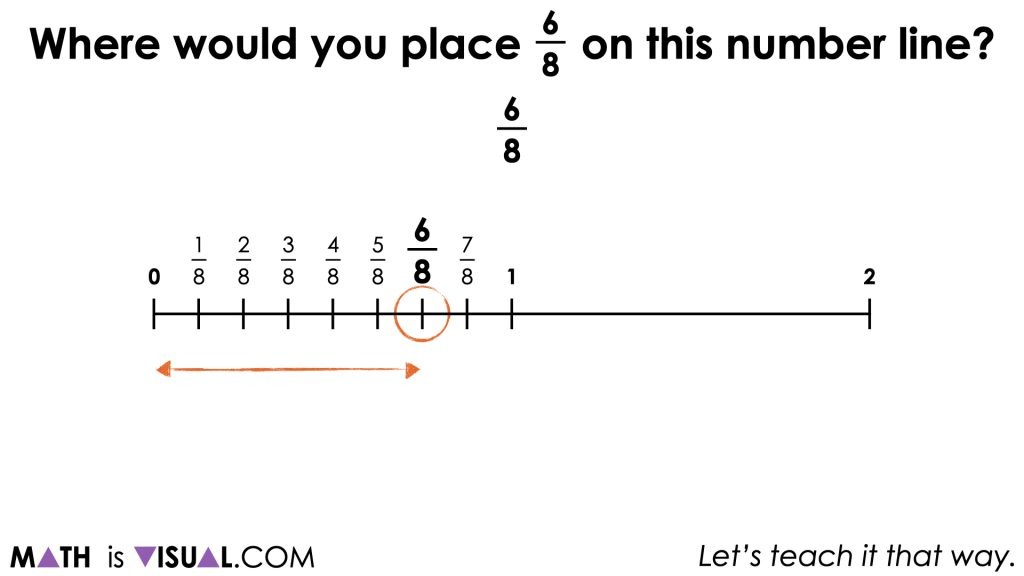Consider asking students to elaborate on what they have ultimately done to determine how they landed on 6 eighths of a metre.

For example, some students might argue that they found 6 groups of half of a half of a half of a half of 2 metres which could be written symbolically as:

6 x (½ of ½ of ½ of ½ of 2)

Are there any other ways that we could represent what was done to partition the line and determine where 6 eighths of a metre would be?

## Want to Explore These Concepts & Skills Further?

Three (3) additional math talk prompts are available in Day 2 of the Wooly Worm Race problem based math unit that you can dive into now.

Why not start from the beginning of this contextual unit of real world lessons from the Make Math Moments Problem Based Units page?

Did you use this in your classroom or at home? How’d it go? Post in the comments!

Math IS Visual. Let’s teach it that way.